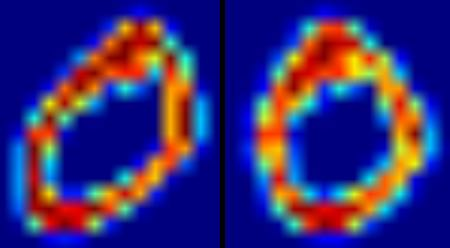# OpenCV-Python 系列 五十五 | 使用OCR手写数据集运行SVM

### 识别手写数字

def deskew(img):
m = cv.moments(img)
if abs(m['mu02']) < 1e-2:
return img.copy()
skew = m['mu11']/m['mu02']
M = np.float32([[1, skew, -0.5*SZ*skew], [0, 1, 0]])
img = cv.warpAffine(img,M,(SZ, SZ),flags=affine_flags)
return imgdef hog(img):
gx = cv.Sobel(img, cv.CV_32F, 1, 0)
gy = cv.Sobel(img, cv.CV_32F, 0, 1)
mag, ang = cv.cartToPolar(gx, gy)
bins = np.int32(bin_n*ang/(2*np.pi))    # quantizing binvalues in (0...16)
bin_cells = bins[:10,:10], bins[10:,:10], bins[:10,10:], bins[10:,10:]
mag_cells = mag[:10,:10], mag[10:,:10], mag[:10,10:], mag[10:,10:]
hists = [np.bincount(b.ravel(), m.ravel(), bin_n) for b, m in zip(bin_cells, mag_cells)]
hist = np.hstack(hists)     # hist is a 64 bit vector
return hist


#!/usr/bin/env python
import cv2 as cv
import numpy as np
SZ=20
bin_n = 16 # Number of bins
affine_flags = cv.WARP_INVERSE_MAP|cv.INTER_LINEAR
def deskew(img):
m = cv.moments(img)
if abs(m['mu02']) < 1e-2:
return img.copy()
skew = m['mu11']/m['mu02']
M = np.float32([[1, skew, -0.5*SZ*skew], [0, 1, 0]])
img = cv.warpAffine(img,M,(SZ, SZ),flags=affine_flags)
return img
def hog(img):
gx = cv.Sobel(img, cv.CV_32F, 1, 0)
gy = cv.Sobel(img, cv.CV_32F, 0, 1)
mag, ang = cv.cartToPolar(gx, gy)
bins = np.int32(bin_n*ang/(2*np.pi))    # quantizing binvalues in (0...16)
bin_cells = bins[:10,:10], bins[10:,:10], bins[:10,10:], bins[10:,10:]
mag_cells = mag[:10,:10], mag[10:,:10], mag[:10,10:], mag[10:,10:]
hists = [np.bincount(b.ravel(), m.ravel(), bin_n) for b, m in zip(bin_cells, mag_cells)]
hist = np.hstack(hists)     # hist is a 64 bit vector
return hist
if img is None:
raise Exception("we need the digits.png image from samples/data here !")
cells = [np.hsplit(row,100) for row in np.vsplit(img,50)]
# First half is trainData, remaining is testData
train_cells = [ i[:50] for i in cells ]
test_cells = [ i[50:] for i in cells]
deskewed = [list(map(deskew,row)) for row in train_cells]
hogdata = [list(map(hog,row)) for row in deskewed]
trainData = np.float32(hogdata).reshape(-1,64)
responses = np.repeat(np.arange(10),250)[:,np.newaxis]
svm = cv.ml.SVM_create()
svm.setKernel(cv.ml.SVM_LINEAR)
svm.setType(cv.ml.SVM_C_SVC)
svm.setC(2.67)
svm.setGamma(5.383)
svm.train(trainData, cv.ml.ROW_SAMPLE, responses)
svm.save('svm_data.dat')
deskewed = [list(map(deskew,row)) for row in test_cells]
hogdata = [list(map(hog,row)) for row in deskewed]
testData = np.float32(hogdata).reshape(-1,bin_n*4)
result = svm.predict(testData)
print(correct*100.0/result.size)


### 练习

1. OpenCV示例包含digits.py，它对上述方法进行了一些改进以得到改进的结果。它还包含参考资料。检查并了解它。